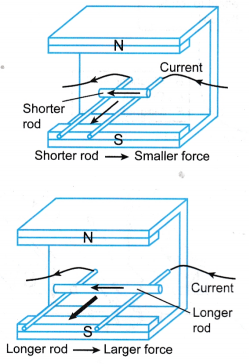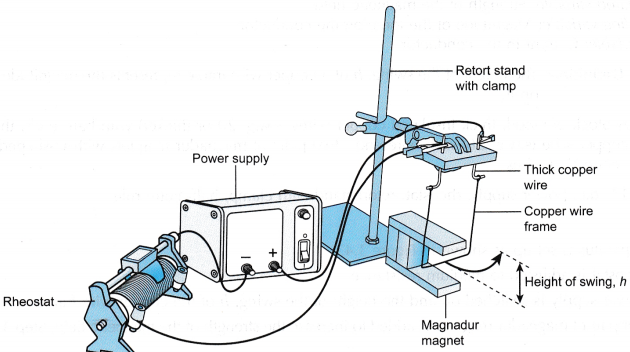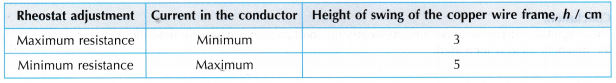## Factors Affecting the Magnitude of the Force on a Current Carrying Conductor

1. The magnitude of the force on a current-carrying conductor in a magnetic field depends on
(a) The current in the conductor,
(b) The strength of the magnetic field.
(c) The length of the conductor in the magnetic field
2. A larger current in the conductor will result in a larger force acting on it if the strength of the magnetic field is kept constant, as shown in Figure.3. The current can be increased by:
(a) Increasing the e.m.f. of the power supply
(b) Using a thicker wire of the same length
(c) Using a shorter wire
4. The magnitude of the force on a current carrying conductor increases when the strength of the magnetic held is increased.
5. A stronger magnetic field can be produced by:
(a) Using more powerful magnets
(b) Using two pairs of magnets with like poles side by side
(c) Placing the magnets closer to each other to narrow the gap between the poles of the magnet
The magnitude of the magnetic force also depends on the length of the wire in the magnetic field. The longer the wire in the magnetic field, the larger the force on the wire as shown in Figure.## Factors Affecting the Magnitude of the Force Experiment

### A. The Current in a Conductor and the Magnitude of the Force

Aim: To investigate the relationship between the current and the magnitude of the force on a conductor.
Problem: What is the relationship between the current and the magnitude of the force on a conductor?
Inference: The current affects the magnitude of the force on a current-carrying conductor.
Hypothesis: The greater the size of the current, the greater the magnitude of the force.
Variables:
(a) Manipulated variable: Current
(b) Responding variable: Magnitude of the force on the conductor
(c) Fixed variable: The strength of the magnetic field
Operational Definition: The height of the swing, h of a copper wire frame represents the magnitude of the force acting on it.
Materials: A block of wood, thick insulated copper wires (s.w.g. 20 or thicker) with bare ends, thin insulated copper wire (s.w.g. 26) with bare ends, a pair of magnadur magnets with U-shaped steel yoke, connecting wires
Apparatus: d.c. power supply, rheostat, retort stand with clamp, half-metre rule
Method:1. The apparatus is set up as shown in Figure.
2. The rheostat is adjusted to maximum resistance so that the current is minimum.
3. The power supply is switched on. The height of the swing, h of the copper wire frame is measured with a half-metre rule.
4. The rheostat is adjusted to minimum resistance so that the current is maximum and step 3 is repeated.

Result:Discusssion:

1. The current in the copper frame produces a magnetic field. The interaction between this magnetic field and the magnetic field from the magnadur magnets produces a force on the copper frame. This force causes the frame to swing upwards.
The height of the swing, h increases when the current in the copper frame is increased.
2. The magnitude of the force increases when the current is increased.

Conclusion:
The magnitude of the force on a current-carrying conductor in a magnetic field increases when the current increases.

### B. The Strength of the Magnetic Field and the Magnitude of the Force

Aim: To investigate the relationship between the strength of the magnetic field and the magnitude of the force on a conductor.
Problem: What is the relationship between the strength of the magnetic field and the magnitude of the force on a conductor?
Inference: The strength of the magnetic field affects the magnitude of the force on a current-carrying conductor.
Hypothesis: The greater the strength of the magnetic field, the greater the magnitude of the force
Variables:
(a) Manipulated variable: Strength of the magnetic field
(b) Responding variable: Magnitude of the force on the conductor
(c) Fixed variable: Current in the conductor
Operational Definition: The height of the swing, h of a copper wire frame represents the magnitude of the force on it.
Materials: A block of wood, thick insulated copper wires (s.w.g. 20 or thicker) with bare ends, thin insulated copper wire (s.w.g. 26) with bare ends, two pairs of magnadur magnets with U-shaped steel yoke, connecting wires
Apparatus: 1 V d.c. power supply, rheostat, retort stand with clamp, half-metre rule
Method:

1. The apparatus is set up as shown in above Figure.
2. The rheostat is adjusted to medium resistance.
3. The power supply is switched on and the height of the swing, h of the copper wire frame is measured.
4. Another pair of magnadur magnets is added to increase the strength of the magnetic field. Step 3 is repeated.

Results:Discussion:

1. The height of the swing, h increases when a stronger magnetic field is applied.
2. The magnitude of the force increases when the strength of the magnetic field is increased.

Conclusion:
The magnitude of the force on a current-carrying conductor in a magnetic field increases when the strength of the magnetic field is increased.